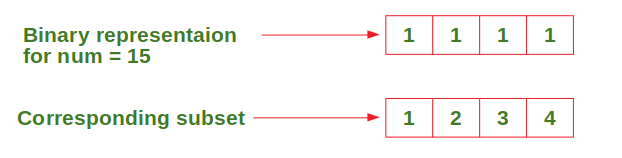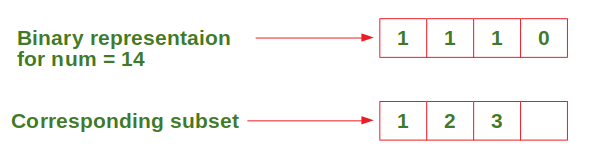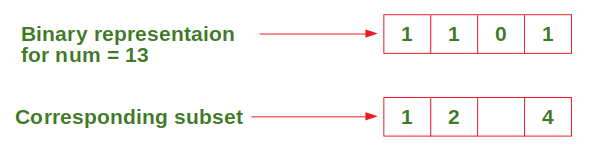# Printing all subsets of {1,2,3,…n} without using array or loop

Given a natural number n, print all the subsets of the setwithout using any array or loop (only the use of recursion is allowed).

Examples:

```Input : n = 4
Output : { 1 2 3 4 }
{ 1 2 3 }
{ 1 2 4 }
{ 1 2 }
{ 1 3 4 }
{ 1 3 }
{ 1 4 }
{ 1 }
{ 2 3 4 }
{ 2 3 }
{ 2 4 }
{ 2 }
{ 3 4 }
{ 3 }
{ 4 }
{ }

Input : n = 2
Output : { 1 2 }
{ 1 }
{ 2 }
{ }
```

## Recommended: Please try your approach on {IDE} first, before moving on to the solution.

Approach:

• Start fromupto 0.
• Consider the binary representation of num with n bits.
• Start from the leftmost bit which represents 1, the second bit represents 2 and so on until nth bit which represents n.
• Print the number corresponding to the bit if it is set.
• Perform the above steps for all values of num until it is equal to 0.

Let’s understand the above approach through an example:

Considering input n = 4, start from.and so on … until num = 0.

Below is the implementation of the above approach:

## C++

 `// C++ code to print all subsets ` `// of {1, 2, 3, n} without using ` `// array or loop, just recursion. ` `#include ` `using` `namespace` `std; ` ` `  `void` `subset(``int``, ``int``, ``int``); ` ` `  `// This recursive function calls subset ` `// function to print the subsets one by one. ` `// numBits --> number of bits needed to ` `// represent the number (simply input value n). ` `// num --> Initially equal to 2 ^ n - 1 and ` `// decreases by 1 every recursion until 0. ` `void` `printSubsets(``int` `numOfBits, ``int` `num)  ` `{ ` `    ``if` `(num >= 0) ` `    ``{ ` `        ``cout << ``"{ "``; ` ` `  `        ``// Print the subset corresponding to ` `        ``// binary representation of num. ` `        ``subset(numOfBits - 1, num, numOfBits); ` `        ``cout << ``"}"` `<< endl; ` ` `  `        ``// Call the function recursively to ` `        ``// print the next subset. ` `        ``printSubsets(numOfBits, num - 1); ` `    ``} ` `    ``else` `        ``return``; ` `} ` ` `  `// This function recursively prints the ` `// subset corresponding to the binary ` `// representation of num. ` `// nthBit --> nth bit from right side ` `// starting from n and decreases until 0 ` `void` `subset(``int` `nthBit, ``int` `num, ``int` `numOfBits) ` `{ ` `    ``if` `(nthBit >= 0)  ` `    ``{ ` `        ``// Print number in given subset only ` `        ``// if the bit corresponding to it ` `        ``// is set in num. ` `        ``if` `(num & (1 << nthBit))  ` `        ``{ ` `            ``cout << numOfBits - nthBit << ``" "``; ` `        ``} ` ` `  `        ``// Check for the next bit ` `        ``subset(nthBit - 1, num, numOfBits); ` `    ``}  ` `    ``else` `        ``return``; ` `} ` ` `  `// Driver Code ` `int` `main() ` `{ ` `    ``int` `n = 4; ` `    ``printSubsets(n, ``pow``(2, n) - 1); ` `} ` ` `  `// This code is contributed by ` `// sanjeev2552 `

## Java

 `// Java code to print all subsets  ` `// of {1, 2, 3, n} without using ` `// array or loop, just recursion. ` `class` `GfG  ` `{ ` ` `  `    ``// This recursive function calls subset ` `    ``// function to print the subsets one by one.  ` `    ``// numBits --> number of bits needed to  ` `    ``// represent the number (simply input value n). ` `    ``// num --> Initially equal to 2 ^ n - 1 and  ` `    ``// decreases by 1 every recursion until 0. ` `    ``static` `void` `printSubSets(``int` `numOfBits, ``int` `num)  ` `    ``{ ` `        ``if` `(num >= ``0``)  ` `        ``{ ` `            ``System.out.print(``"{ "``); ` `             `  `            ``// Print the subset corresponding to  ` `            ``// binary representation of num. ` `            ``subset(numOfBits - ``1``, num, numOfBits); ` `            ``System.out.println(``"}"``); ` `             `  `            ``// Call the function recursively to  ` `            ``// print the next subset. ` `            ``printSubSets(numOfBits, num - ``1``); ` ` `  `        ``} ``else` `            ``return``; ` `    ``} ` ` `  `    ``// This function recursively prints the  ` `    ``// subset corresponding to the binary  ` `    ``// representation of num. ` `    ``// nthBit --> nth bit from right side  ` `    ``// starting from n and decreases until 0. ` `    ``static` `void` `subset(``int` `nthBit, ``int` `num, ``int` `numOfBits)  ` `    ``{ ` `        ``if` `(nthBit >= ``0``)  ` `        ``{ ` `            ``// Print number in given subset only ` `            ``// if the bit corresponding to it  ` `            ``// is set in num. ` `            ``if` `((num & (``1` `<< nthBit)) != ``0``) ` `            ``{ ` `                ``System.out.print(numOfBits - nthBit + ``" "``); ` ` `  `            ``} ` `             `  `            ``// Check for the next bit  ` `            ``subset(nthBit - ``1``, num, numOfBits); ` `        ``} ``else` `            ``return``; ` `    ``} ` `     `  `    ``// Driver code ` `    ``public` `static` `void` `main(String[] args)  ` `    ``{ ` `        ``int` `n = ``4``; ` `        ``printSubSets(n, (``int``) (Math.pow(``2``, n)) -``1``); ` `    ``} ` `} ` ` `  `// This code is contributed by laststringx `

## Python3

 `# Python3 code to print all subsets  ` `# of {1, 2, 3, …n} without using ` `# array or loop, just recursion. ` ` `  `# This recursive function calls subset ` `# function to print the subsets one by one.  ` `# numBits --> number of bits needed to  ` `# represent the number (simply input value n). ` `# num --> Initially equal to 2 ^ n - 1 and  ` `# decreases by 1 every recursion until 0. ` `def` `printSubsets(numOfBits, num): ` `     `  `    ``if` `num >``=` `0``: ` `        ``print``(``"{"``, end ``=` `" "``) ` ` `  `        ``# Print the subset corresponding to  ` `        ``# binary representation of num. ` `        ``subset(numOfBits``-``1``, num, numOfBits) ` `        ``print``(``"}"``) ` ` `  `        ``# Call the function recursively to  ` `        ``# print the next subset. ` `        ``printSubsets(numOfBits, num``-``1``) ` `         `  `    ``else``: ` `        ``return` ` `  `# This function recursively prints the  ` `# subset corresponding to the binary  ` `# representation of num. ` `# nthBit --> nth bit from right side  ` `# starting from n and decreases until 0. ` `def` `subset(nthBit, num, numOfBits): ` `     `  `    ``if` `nthBit >``=` `0``: ` `         `  `        ``# Print number in given subset only ` `        ``# if the bit corresponding to it  ` `        ``# is set in num. ` `        ``if` `num & (``1` `<< nthBit) !``=` `0``: ` `            ``print``(numOfBits ``-` `nthBit, end ``=` `" "``) ` `         `  `        ``# Check for the next bit  ` `        ``subset(nthBit``-``1``, num, numOfBits) ` `         `  `    ``else``: ` `        ``return` ` `  `# Driver Code     ` `n ``=` `4` `printSubsets(n, ``2``*``*``n ``-` `1``) `

## C#

 `// C# code to print all subsets  ` `// of {1, 2, 3, n} without using  ` `// array or loop, just recursion. ` `using` `System; ` ` `  `class` `GfG  ` `{  ` ` `  `    ``// This recursive function calls subset  ` `    ``// function to print the subsets one by one.  ` `    ``// numBits --> number of bits needed to  ` `    ``// represent the number (simply input value n).  ` `    ``// num --> Initially equal to 2 ^ n - 1 and  ` `    ``// decreases by 1 every recursion until 0.  ` `    ``static` `void` `printSubSets(``int` `numOfBits, ``int` `num)  ` `    ``{  ` `        ``if` `(num >= 0)  ` `        ``{  ` `            ``Console.Write(``"{ "``);  ` `             `  `            ``// Print the subset corresponding to  ` `            ``// binary representation of num.  ` `            ``subset(numOfBits - 1, num, numOfBits);  ` `            ``Console.WriteLine(``"}"``);  ` `             `  `            ``// Call the function recursively to  ` `            ``// print the next subset.  ` `            ``printSubSets(numOfBits, num - 1);  ` ` `  `        ``} ``else` `            ``return``;  ` `    ``}  ` ` `  `    ``// This function recursively prints the  ` `    ``// subset corresponding to the binary  ` `    ``// representation of num.  ` `    ``// nthBit --> nth bit from right side  ` `    ``// starting from n and decreases until 0.  ` `    ``static` `void` `subset(``int` `nthBit, ``int` `num, ``int` `numOfBits)  ` `    ``{  ` `        ``if` `(nthBit >= 0)  ` `        ``{  ` `            ``// Print number in given subset only  ` `            ``// if the bit corresponding to it  ` `            ``// is set in num.  ` `            ``if` `((num & (1 << nthBit)) != 0)  ` `            ``{  ` `                ``Console.Write(numOfBits - nthBit + ``" "``);  ` ` `  `            ``}  ` `             `  `            ``// Check for the next bit  ` `            ``subset(nthBit - 1, num, numOfBits);  ` `        ``} ``else` `            ``return``;  ` `    ``}  ` `     `  `    ``// Driver codeM ` `    ``public` `static` `void` `Main(String[] args)  ` `    ``{  ` `        ``int` `n = 4;  ` `        ``printSubSets(n, (``int``) (Math.Pow(2, n)) -1);  ` `    ``}  ` `} ` ` `  `// This code is contributed by Srathore `

Output:

```{ 1 2 3 4 }
{ 1 2 3 }
{ 1 2 4 }
{ 1 2 }
{ 1 3 4 }
{ 1 3 }
{ 1 4 }
{ 1 }
{ 2 3 4 }
{ 2 3 }
{ 2 4 }
{ 2 }
{ 3 4 }
{ 3 }
{ 4 }
{ }
```

Time Complexity:Attention reader! Don’t stop learning now. Get hold of all the important DSA concepts with the DSA Self Paced Course at a student-friendly price and become industry ready.

My Personal Notes arrow_drop_upI like solving puzzles

If you like GeeksforGeeks and would like to contribute, you can also write an article using contribute.geeksforgeeks.org or mail your article to contribute@geeksforgeeks.org. See your article appearing on the GeeksforGeeks main page and help other Geeks.

Please Improve this article if you find anything incorrect by clicking on the "Improve Article" button below.